# Subtraction + reason - math problems

#### Number of problems found: 52

• When 5When 5 is subtracted from both my numerator and denominator I become 3/4. What fraction i am?
• Petra's testThe answer will be given 8 marks, and the wrong answer will be deducted 3 marks. The total number of marks obtained by the school is 36. If Petra answered six questions correctly,   how many questions were answered incorrectly by the student from the scho
• Row for lunchThere are less than 66 people in a row for lunch. In front of Eva, there are 28 students. Behind Martin, there are 30 people. Between Eva and Martin, there are 17 people. How many students stand behind Eva if Martin stands in front of Eva?
• Metal ballsFour metal balls with a diameter of 5 cm are placed in a measuring cylinder with an inner diameter of 10 cm. What is the smallest water volume to be poured into the cylinder so that all balls are below the water level?
• Ground levelThe temperature at ground level in Helsinki is 20°C lower than in London. The temperature in a basement flat in Helsinki is 5°C below the temperature at ground level. If it is -29°c in the basement, what is the temperature in London?
• Six-eightsSix-eights of the one hundred pupils joined the Math Glee club. If the Math Glee club members were grouped into three, how many members were in each group?
• GranddaughterIn 2014, the sum of the ages of Meghan's aunt, her daughter and her granddaughter was equal to 100 years. In what year was the granddaughter born, if we know that the age of each can be expressed as the power of two?
• DodecagonCalculate the size of the smaller of the angles determined by lines A1 A4 and A2 A10 in the regular dodecagon A1A2A3. .. A12. Express the result in degrees.
• A farmer's heritage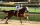A farmer died leaving his 17 horses to his 3 sons. When his sons opened up the Will it read: My eldest son should get 1/2 (half) of total horses; My middle son should be given 1/3rd (one-third) of the total horses; My youngest son should be given 1/9th (o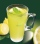In January, 2 liters of lemonade were sold for CZK 24, while in February, 2.5 liters of lemonade sold at same price. How much percent was 1 liter of lemonade cheaper in February than in January?
• Math testObelix filled a mathematical test in which he answered 25 questions. For every correct answer, he received 5 points, for each bad answer he had 3 points deducted. Obelix gained 36% of all points in the test. How many questions did he solve correctly?
• The HotelThe Holiday Hotel has the same number of rooms on each floor. Rooms are numbered with natural numerals sequentially from the first floor, no number is omitted, and each room has a different number. Three tourists arrived at the hotel. The first one was in
• Game 27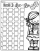Susan wanted to play the game. In the beginning, the first says a number from 1 to 8. Then the second player adds a number from 1 to 5 and tells the sum. Again, the Susan adds a number from 1-5 and say sum and etc. . . The winner must say the number 27. W
• The Stolen Money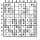A man walks into a store and steals a \$100 bill. 5 minutes later, he returns to the store and buys stuff worth \$70. He pays with the bill that he had stolen, so the owner of the store returns him \$30. How many dollars did the store owner lose?
• In the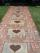In the garden, workers will pave a 1-meter-wide sidewalk with tiles around the block-shaped pool. The dimensions of the bottom of the pool are 8.5 meters and 6 meters. The height of the pool walls is 2 meters. How many m2 of pavement will be laid with til
• The coilHow many ropes (the diameter 8 mm) fit on the coil (threads are wrapped close together) The coil has dimension: the inner diameter 400mm, the outside diameter 800mm and the length of the coil is 470mm
• Identity123456789 = 100 Use only three plus or minus characters to correct previous identity/equation.
• Princess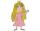Princess has lost 321 beads and Prince George are all trying to find. On the first day he found all but 83 beads. On the second day he found 68 beads. On the third day he found the rest, but the princess had lost 76. How many beads had Princess after theThere were a total of 200 cookies in the box. They used sugar and chocolate icing in their production. They used chocolate icing for 157 biscuits. They used sugar icing for 100 biscuits. How many of these cookies have both toppings?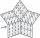A five-digit odd number has the sum of all digits (digits) five and contains two zeros. If we move each digit in the number one place to the left and move the first digit to the last place, we get a number 20,988 smaller. Find this unknown five-digit numb